# Logistic Map equation: A Simple Equation with Enigmatic Implications

June 22, 2021

By  Soumya Shaw

`lang: en_US`

## Introduction

It's been centuries now since humans have walked through the path of Intelligence to know the intricacies of numbers, delved deeper into reasoning, and found pivotal paradigms that have set the course of evolution. The inquisitiveness of the human mind has fueled this long journey of technological advancements, and we continue to do the same unfurling even more complex mysteries of nature. Mathematics and Physics have been at the forefront, spearheading the improvements. Knowing it all, it is true that if you don't find Mathematics beautiful, it is because you haven't explored it enough. It is pretty fascinating to even think of how the universe works with a few well-defined equations and transcends into something so grand and elegant. This blog discusses a similar sleek yet straightforward case study of an equation that proved to comply in unexpected ways.

## The Equation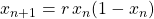An absurd title, right? If an equation is simple, how can it produce complex outcomes? Well, let me take this opportunity to break this stereotype of Mathematics! Have a good look at the equation and note how it has no Trigonometric term, no differential operator, and not at all involve any complex Math like the Schrodinger equation. You might have heard about the famous Butterfly effect as well, and let me unfurl a hint about it as well - this equation is capable of eliciting the phenomenon of the mentioned effect. So, from where in the equation does the complexity come, exactly? Worry not; we will get all the answers ourselves once we dive deep into the equation.Relationship of Logistic Map Equation without 'r'

The logistic map equation represents a discrete relationship between the current value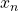and its future value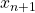. The reader might have a propensity to believe that the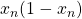term may be the real culprit rendering the complexity. However, combining the regeneration rate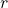and connecting the future term with the current term actually generates the platform for the complexity. Without them, the resultant graph would be just like the Figure shown. The whole progression depends on the initial population and the regeneration rate. Let us run a code and visualize how these two parameters affect the progression intuitively.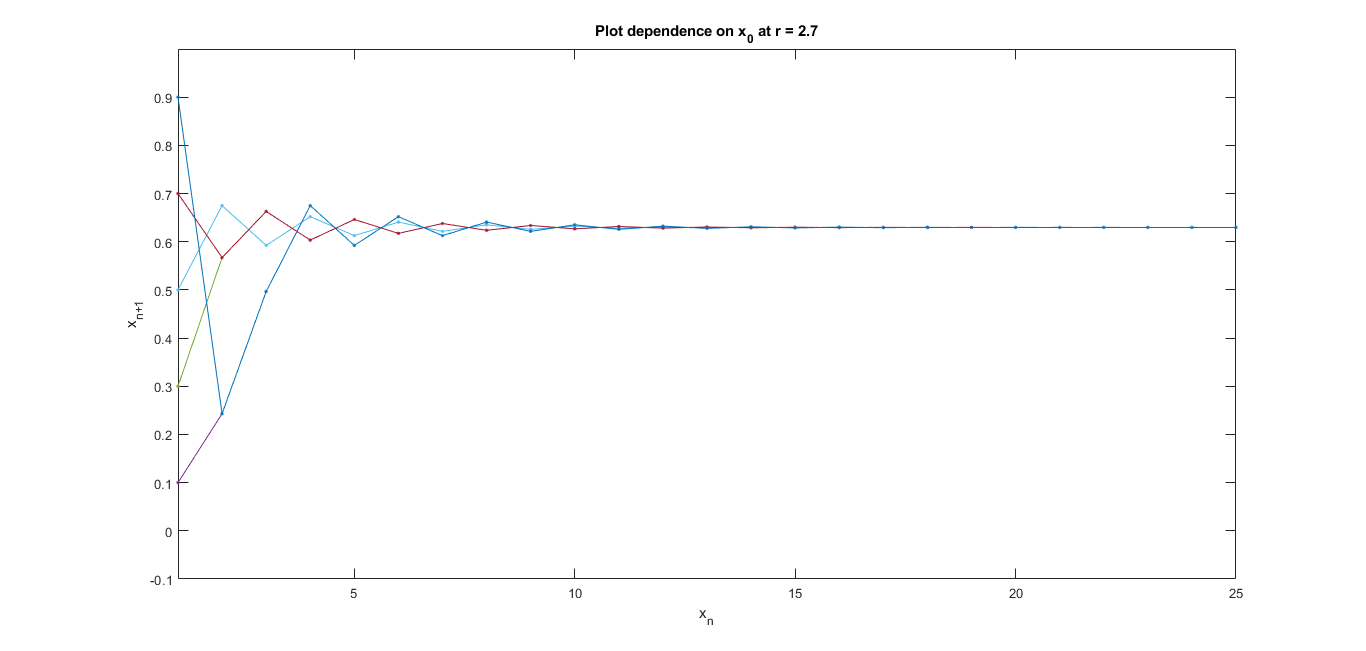Dependence of series onAs clearly evident from the output graphs, the overall pattern is unaffected by the change in the initial value. Instead, it just wavers the plot for the first few generations in the y-axis before converging to the same value for a given value of.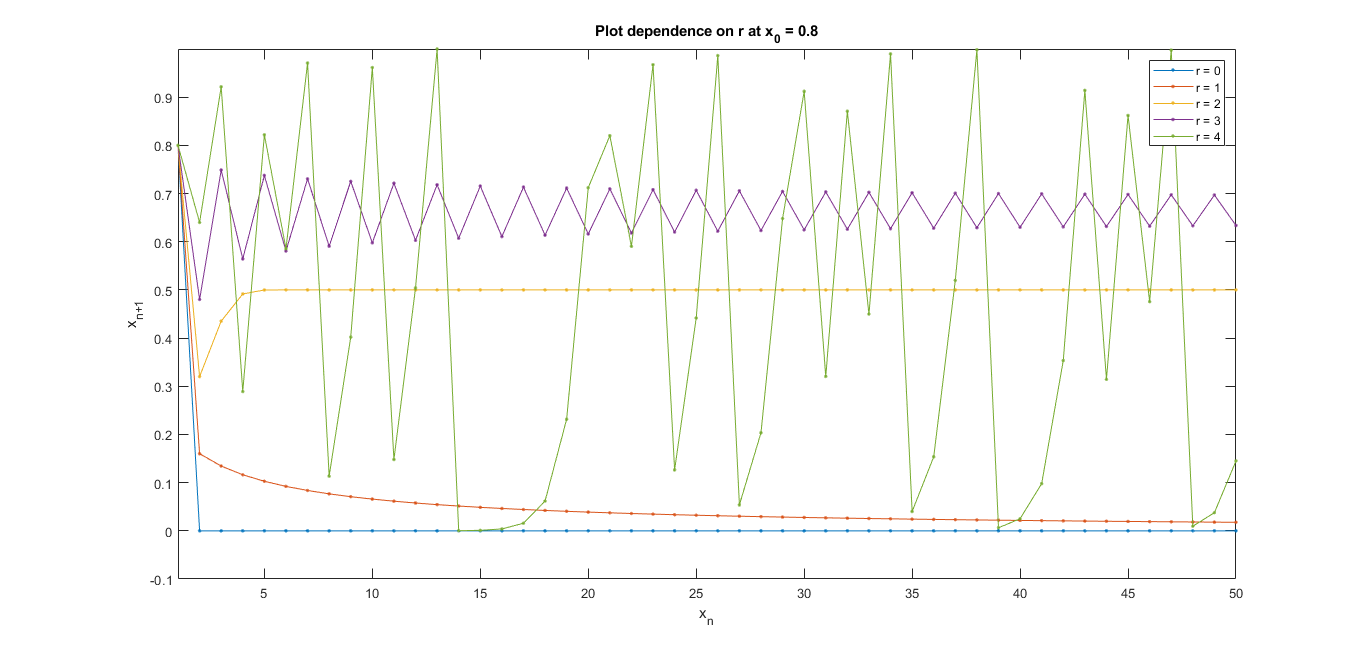Dependence of series onOn the other hand, varying theengenders the actual pattern of contemplation. At lower values of, the system converges to a value after few generations. We will see a different way to visualize that shortly. Still, the central eureka moment lies when the graph doesn't show convergence for values ofhigher than 2, still showing a periodic pattern. At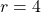, the plot gets devoid of any such apparent periodicity. It looks like a random sequence fluctuating without any pattern being followed. In the mathematical domain, we term the system to be chaotic.

Let us iterate through some of the applications of this equation before connecting it to the Butterfly effect. Have you ever wondered about the Beatles in your nearest park? Have you observed how the population might behave in an environment like that? Assume there is an initial population of beetles that arrived in a garden.  As we may predict, their population in the next generation will be dependent on the previous generation, and the regeneration rate will determine how fast or slow their population grows or diminishes. Any such system will lead to an infinite population with subsequent generations, but that is not the case we encounter. We add the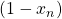term for the next generation's population that serves as the negative feedback loop and checks that growth to infinity. Now, we observe the pattern that emulates the actual population of Beatles over generations. When the populations of beetles grow exceedingly high, the resources fall short, and the population drops sharply next generation. After few generations of fluctuations, the Beatles settle their population, adapting to the resources available. If the sources are well suited, the converged population is higher or the other way around. However, some patterns seem to repeat instead of converging. We observe most biological systems like trees, producing an increased number of fruits one year and lower the next. This phenomenon is known as biennial bearing. Many kinds of research support this pattern that has been officially published over time.

Not even abiological systems are spared. For example, water dripping through a tap is observed to follow a periodic cycle. Some follow single time intervals, some repeat a cycle of two-time intervals, some even higher-order cycles, and some just go on to show chaotic response, following no observable pattern. It is astonishing to know that a single equation can represent all these conditions by varying a single parameter.

You might remember when some numerical in Physics gave out answers like 20.069. We carelessly concluded the answer to be 20, stating it to be approximate in brackets, and that wasn't wrong either. After all, what change can it bring with the excess 0.069, anyway? However, nature taught us that even rounding off by 0.00001 can lead to wild implications in the long run. Edward Lorenz once, trying to model weather patterns through computers, mistakenly rounded off some initial values at 4th or 5th decimal place and allowed the system to progress over time. He was later shocked to find the frantic divergence of predictions from the case in which he didn't round off the initial conditions. It seems nature doesn't round off values for simplicity. This particular effect came to known as the Butterfly effect, and its popularity is known to you. Enough stories! It's time to put our coding knowledge into practice (code 4). Taking the same Logistic Map equation, we differ the initial conditions just by 0.0001 to see the progression of the output sequence.

clc
clear all
close all
%% CODE 1
x = 0:0.01:1;
y = x.*(1-x);
figure(1)
plot(x,y); % plotting the graph
title("x_{n+1} Vs x_n Relationship")
xlabel("x_n") % Generation
ylabel("x_{n+1}") % Population growth
axis([0 1 0 0.5]) % setting axis range
%% CODE 2
x_0 = 0.1:0.2:1;
r = 2.7;
x = [];
for i=1:length(x_0)
x(i,1) = x_0(i); % assigning initial population
end
for i=2:25
for j=1:length(x_0)
x_n = r*x(j,i-1)*(1-x(j,i-1)); % logistic map
x(j,i) = x_n;
figure(2)
plot(x(j,:),'.-')
axis([1 25 -0.1 1])
hold on
end
%pause(0.1);
end
title("Plot dependence on x_0 at r = 2.7")
xlabel("x_n") % Generation
ylabel("x_{n+1}") % Population growth
hold on
%% CODE 3
x_0 = 0.8;
r = 0:4;
x = [];
for i=1:length(r)
x(i,1) = x_0; % assigning initial population
end
for i=1:length(r)
for j=2:50
x_n = r(i)*x(i,j-1)*(1-x(i,j-1)); % logistic map
x(i,j) = x_n;
end
figure(3)
plot(x(i,:),'.-')
hold on
end
axis([1 50 -0.1 1])
title("Plot dependence on r at x_0 = 0.8")
xlabel("x_n") % Generation
ylabel("x_{n+1}") % Population growth
legend('r = 0','r = 1','r = 2','r = 3','r = 4')
%% CODE 4
x_0 = [0.8 0.80001 0.80002 0.80003 0.80004];
r = 3.9;
x = [];
for i=1:length(x_0)
x(i,1) = x_0(i);
end
for i=2:50
for j=1:length(x_0)
x_n = r*x(j,i-1)*(1-x(j,i-1)); % logistic map
x(j,i) = x_n;
figure(4)
plot(x(j,:),'.-')
axis([1 50 -0.1 1])
hold on
end
end
title("Visualization of Butterfly effect")
xlabel("x_n") % Generation
ylabel("x_{n+1}") % Population growth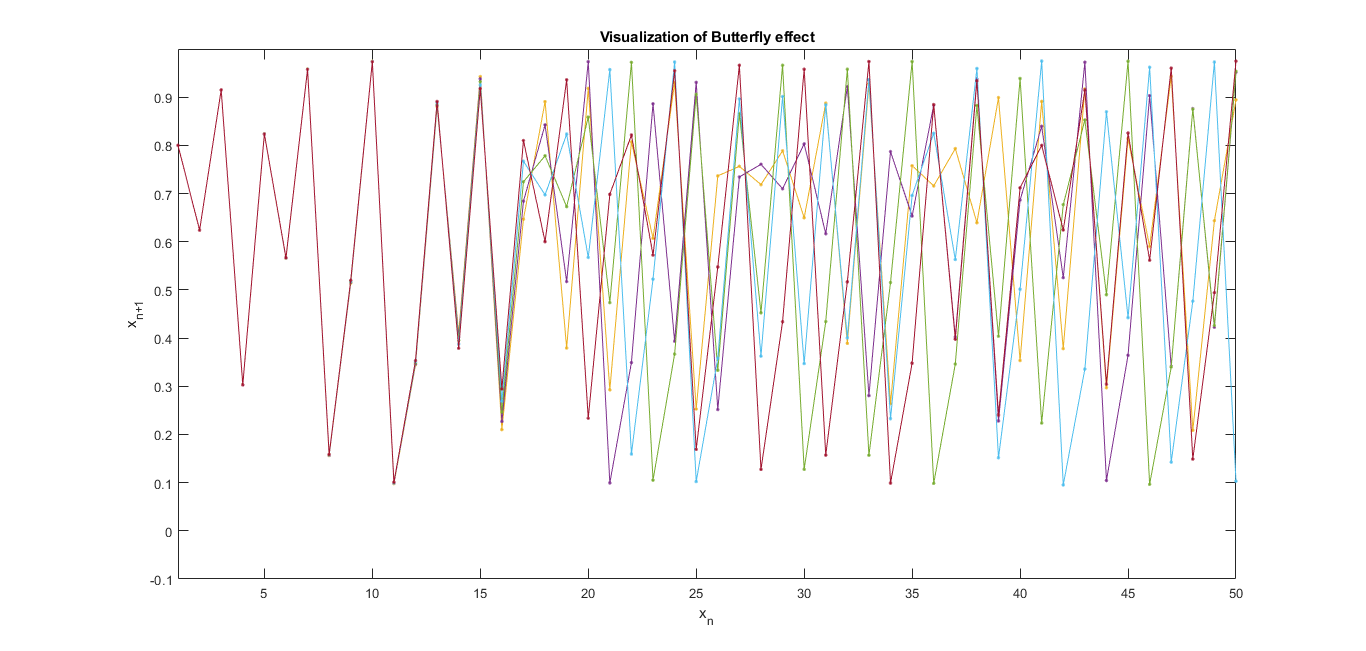The Butterfly Effect

As I mentioned, we expected that! Even though the initial population was just off by 0.0001 from each other, twenty generations later, nobody can deduce any possible correlation between each progression. That's the beauty of the Butterfly effect! Well, only if you don't get overwhelmed by it.

## Chaos Theory

“As far as the laws of Mathematics refer to reality, they are not certain, and as far as they are certain, they do not refer reality.”
- Einstein

You might remember me mentioning the beauty of math and describing physical systems just with few well-defined systems. That was the golden rule that the pioneers of Science till Lorenz followed. Then however, the notion shifted to a new branch of Mathematics, advocating the uncertainties entangled in every aspect of predictions that soon came to be known as the Chaos Theory. The theory demonstrated how systems with deterministic nature could lead to unpredictability. The path of prophecy is hazy and blurry after a specific limit. Knowing that there is a limit to the certainty of measurement, we cannot predict behaviors accurately till infinite time.

## Uniformity of the Randomness

The logistic map equation was once used for pseudo-random number generators in computers. It was the most apparent method to generate something unpredictable on a deterministic computer. I specifically mentioned pseudo-random numbers since knowing the initial condition for the generator still gives a deterministic pattern in hand, and you can imitate the same sequence that we got earlier. Although determining the initial conditions is not the most straightforward task to perform, we already showed you how being off by just 0.0001 gets you nowhere near the former. So, let's plot a sequence of randomness given by the logistic map and a current random number generator using code 5.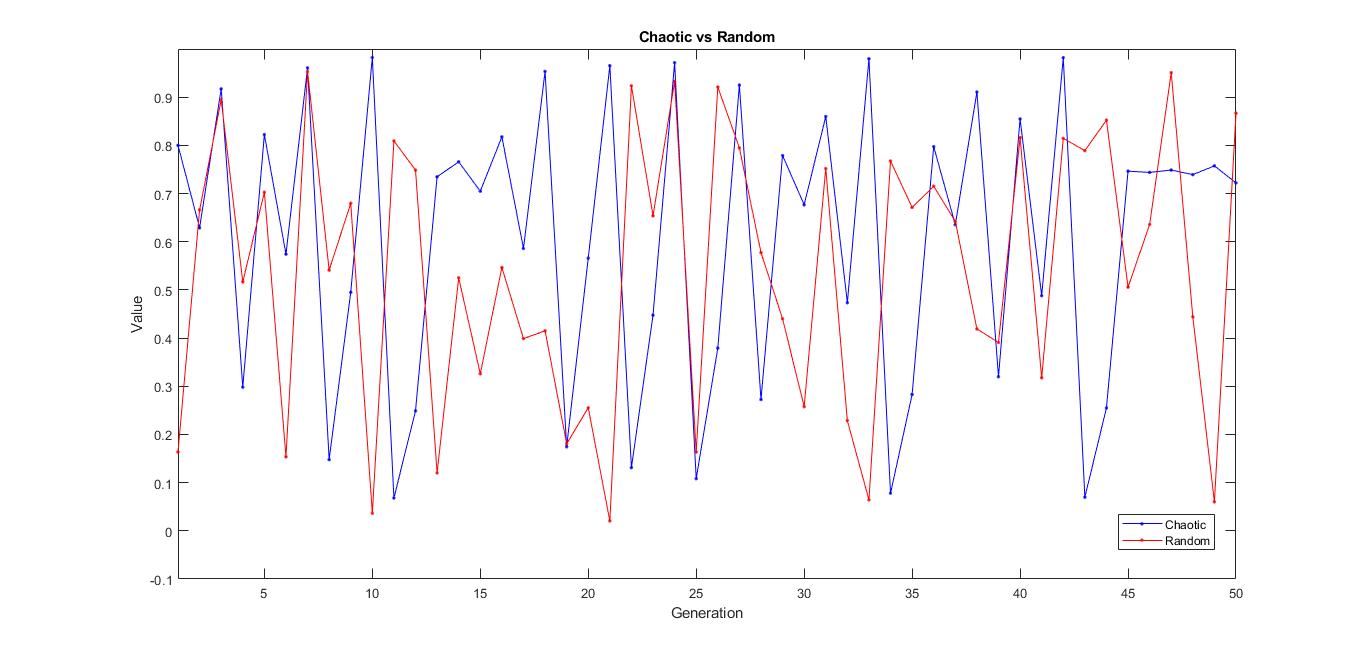Randomness vs Chaotic Sequence

The emergent graph doesn't show any noticeable feature between them. Is there something concealed deep within? Let's plot in a different frame and experiment. We intend to plot the Poincaré plot and reflect the self-similarity property of a sequence.

clc
clear all
close all
%% CODE 5
x_0 = 0.8;
r = 3.9;
x = [x_0]; % assigning initial value
for i=2:50
x_n = r*x(i-1)*(1-x(i-1));
x(i) = x_n;
end
s = rand(50,1)';
figure(2)
plot(x,'b.-')
hold on
plot(s, 'r.-')
axis([1 50 -0.1 1])
title("Chaotic vs Random")
xlabel("Generation")
ylabel("Value")
legend('Chaotic','Random')
%% CODE 6
x_0 = 0.8;
r = 3.9;
x = [x_0]; % assigning initial value
for i=2:500
x_n = r*x(i-1)*(1-x(i-1));
x(i) = x_n;
end
s = rand(500,1)';
s1 = s(2:499);
s2 = s(3:500);
s = s(1:498);
x1 = x(2:499);
x2 = x(3:500);
x = x(1:498);
figure(3)
plot3(x,x1,x2,'.')
xlabel('x_n')
ylabel('x_{n+1}')
zlabel('x_{n+2}')
grid on
hold on
plot3(s,s1,s2,'.')
title('Poincare Plot')
legend('Chaotic','Random');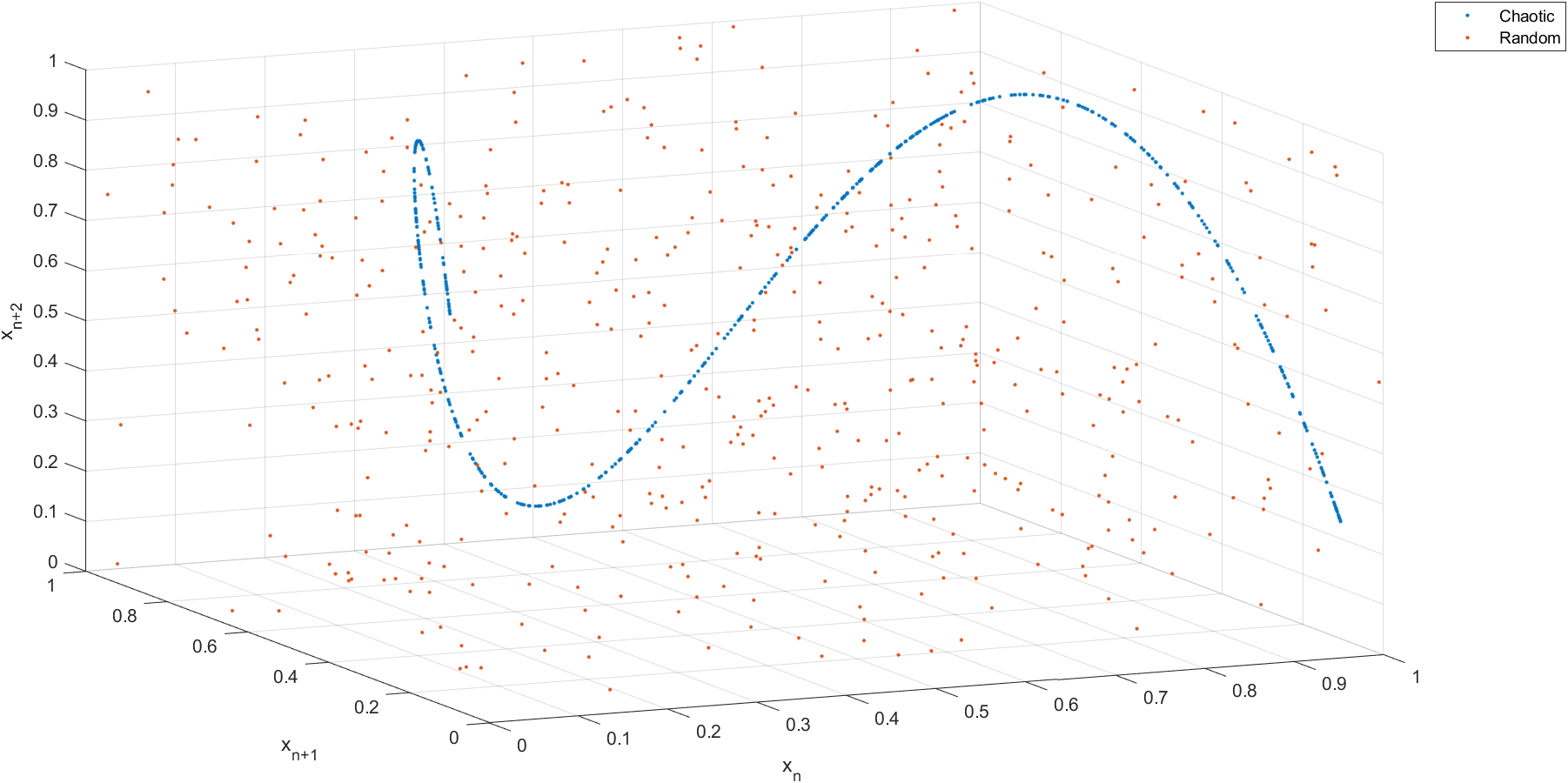Poincare Plot of two sequences

The outcome explicates a significant insight into the true nature of the pseudo-random sequence and its distinguishability compared with a random series. The random sequence, as you can infer, is scattered through space and signifies no deducible pattern. In contrast, if you look close enough, you'll find the first graph that we witnessed in this article to be precisely similar to this graph, emerging from the X-Y plane.

## Bifurcation and Period Doubling

Another type of plot known as the Coweb plot helps us reference the convergence value the sequence is chasing with a visual aid. The blue line in the Coweb plot is represented by the logistic map equation, and the red line is just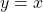plot. The plot starts from the initial population from the x-axis. It progresses vertically till it encounters the point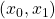. Consequently, it progresses towards the red line until it touches the line and starts taking the reached point as the initial population. Evidently, the series always tries to reach the intersection point of the logistic map equation andand serves as the great attractor; Nevertheless, not all progressions reach their fate and wander around following the stated algorithm trying to get closer but generating a chaotic system at best.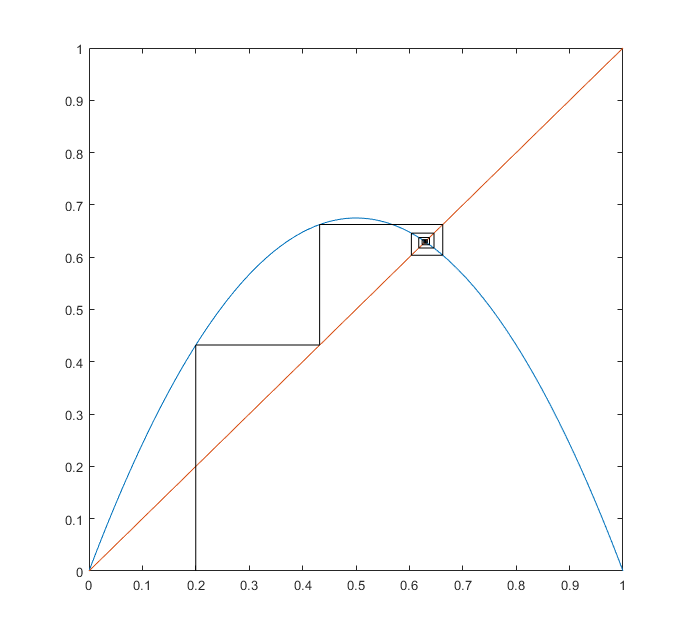Coweb plot

We move on to the last discernible insight that we can find from the equation we have been dealing with. We have plotted the sequence in the discrete series in generation form over and over again. But we have not yet focused on the convergence value for the variable. So, let's plot that as well (code 8). You can try it using the code as I simultaneously narrate what we are up to. For lower values of, where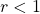, the regeneration rate symbolizes unsuitable conditions for growth. Hence the population dies out, converging to the value of zero. As we go higher, we see the convergence value rising higher until we reach, and we observe a bifurcation in the convergence. No matter how long we let the series progress, there is no single value it converges to. The graph further bifurcates into 4 convergence values around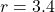. This trend continues for a while, doubling the period each successive time we let thevalue increase. Starting from 1, it goes to 2, 4, 8, 16, and consequently right into chaos. It looks like a maze with no apparent end to the period of repetition. Now you can perfectly relate to how just varying the regeneration rate at specific values emulate the exact patterns we see in nature. Let it be the population of insects, dripping water from a tap, or such similar examples. Crevier et. al. recorded the same period doubling pattern in the flickering of eyes in a salamander when light pulses with increasing frequency are shone over their eyes. You have a look at the results as well.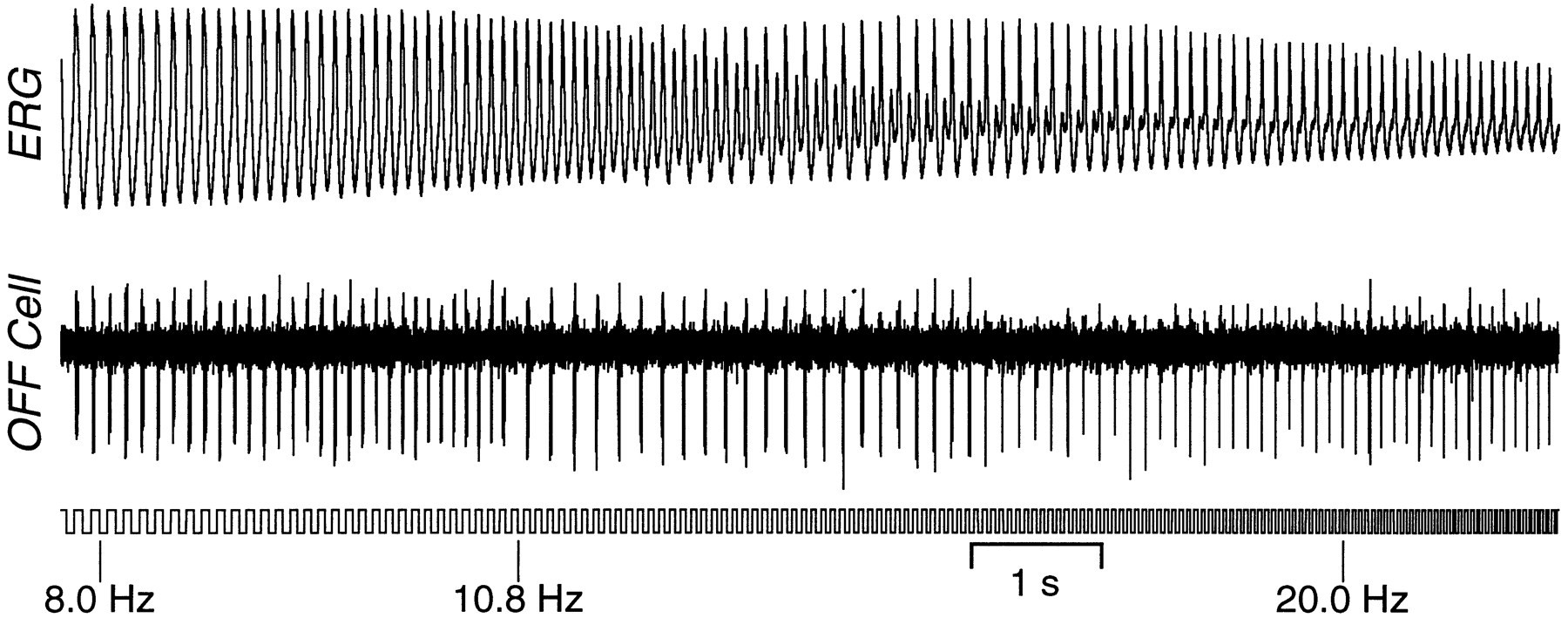Pattern of flickering of eyes in a Salamander

clc
clear all
close all
%% CODE 7
r = 2;
x = 0:0.01:1;
fy = r*x.*(1-x); % logistic map
plot(x,fy);
hold on
plot(x,x); % straight line through origin
hold on
x(1) = 0.2;
plot([x(1),x(1)],[0,x(1)],'black');
hold on
for i=1:100
x(i+1)= r*x(i)*(1-x(i)); % logistic map series progression
figure(1)
plot([x(i),x(i)],[x(i),x(i+1)],'black');
pause(0.05)
hold on
h=plot([x(i),x(i+1)],[x(i+1),x(i+1)],'black');
pause(0.05)
hold on
end
%% CODE 8
x_0 = 0.8;
r = 0:0.01:4;
x = [];
for i=1:length(r)
x(i,1) = x_0;
end
for i=2:300 % count series values upto 300 generations
for j=1:length(r)
x_n = r(j)*x(j,i-1)*(1-x(j,i-1));
x(j,i) = x_n;
if i>100 % discard first 100 generations
figure(2)
plot(r(j),x(j,i),'r.')
end
hold on
end
end
title("Bifurcation & Period Doubling")
xlabel("Regeneration rate 'r'")
ylabel("Convergence value of Sequence")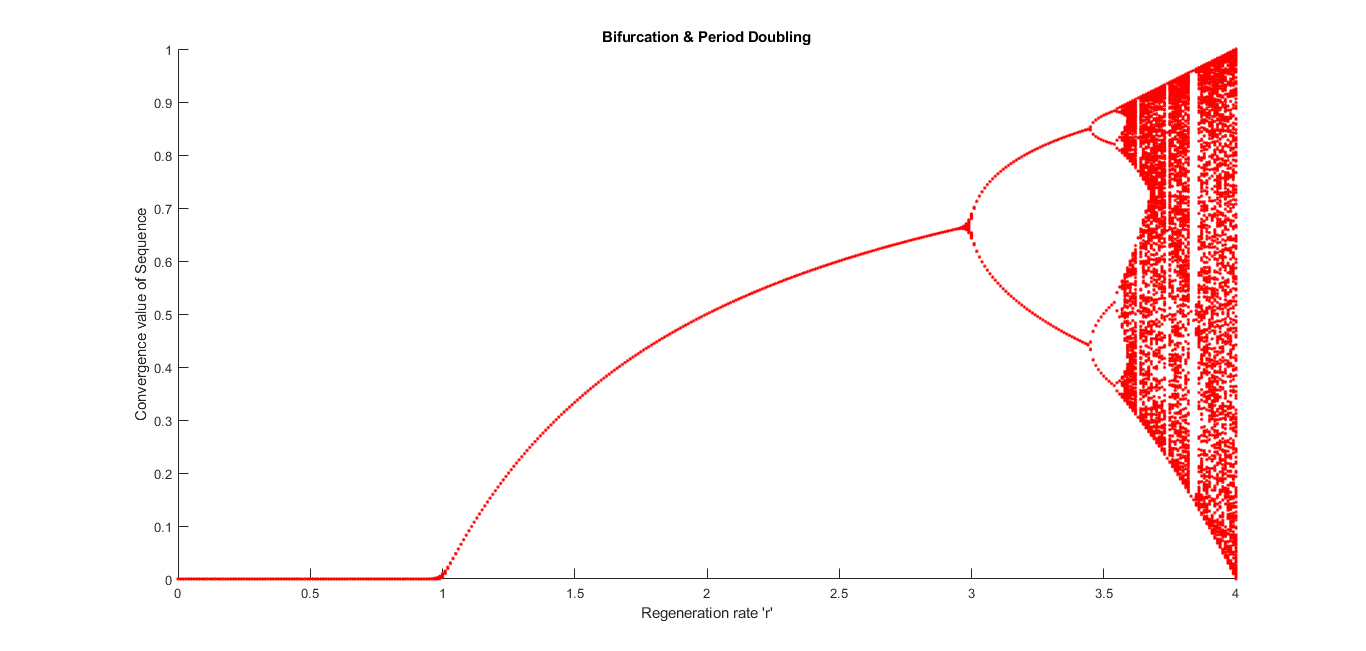Bifurcation Diagram

## Conclusion

Nature is in itself the most complex thing to simulate even after simplification. It has its own way of progression; it just couldn't care if it's simple to understand or not. It is our evolution of Intelligence that enabled us to see the complexity ingrained within physical processes. It is better not to suppress our silly doubts and let them out since the butterfly effect teaches us that "a small wish can grow into great outcomes." Do let me know how interesting the equation proved to be and in what ways do you feel it applies to even more real-life scenarios or any other insights we can draw from them.

Ready to take your MATLAB skills to the next level? Look no further! At MATLAB Helper, we've got you covered. From free community support to expert help and training, we've got all the resources you need to become a pro in no time. If you have any questions or queries, don't hesitate to reach out to us. Simply post a comment below or send us an email at [email protected].

And don't forget to connect with us on LinkedIn, Facebook, and Subscribe to our YouTube Channel! We're always sharing helpful tips and updates, so you can stay up-to-date on everything related to MATLAB. Plus, if you spot any bugs or errors on our website, just let us know and we'll make sure to fix it ASAP.

Ready to get started? Book your expert help with Research Assistance plan today and get personalized assistance tailored to your needs. Or, if you're looking for more comprehensive training, join one of our training modules and get hands-on experience with the latest techniques and technologies. The choice is yours – start learning and growing with MATLAB Helper today!

Education is our future. MATLAB is our feature. Happy MATLABing!

#### Soumya Shaw

Soumya Shaw is currently a graduated B.Tech student in Electronics & Communication from the Vellore Institute of Technology. He is an IEEE & SGAC member and interestingly working on Machine Learning, Image Processing and Digital Signal Processing. Besides, he is overzealous in the field of Cosmology, Higher Dimensional Mathematics & Quantum Mechanics.

•It is the perfect time to make some plans for the future and it is time to be happy. I’ve read this post and if I could I desire to suggest to you some interesting things or suggestions. Perhaps you could write the next articles referring to this article. I want to read more things about it!
logistics

•Syed Zubair shah says:

Can you tell me how can i make lyapunov exponent of sin(x) ?

#### MATLAB Helper ®

Use Website Chat or WhatsApp at +91-8104622179